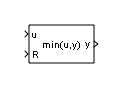MinMax Running Resettable

Determine minimum or maximum of signal over time

• Library:

•Description

The MinMax Running Resettable block outputs the minimum or maximum of all past inputs u. You specify whether the block outputs the running minimum or maximum with the Function parameter.

The block can reset its state based on an external reset signal R. When the reset signal R is nonzero (true), the block resets the output to the value of the Initial condition parameter.

The input can be a scalar, vector, or matrix signal. The block outputs a signal having the same dimensions as the input. Each output element equals the running minimum or maximum of the corresponding input elements.

Ports

Input

expand all

Input signal as a scalar, vector, or matrix. Based on what you specify for the Function parameter, the block outputs the minimum or maximum value of all past inputs u.

If you specify a scalar value for the Initial condition parameter, the block expands the parameter to have the same dimensions as nonscalar input u.

Data Types: single | double | int8 | int16 | int32 | int64 | uint8 | uint16 | uint32 | uint64 | Boolean | fixed point | bus

The input port accepting the reset signal as a scalar, vector, or matrix. When the reset signal is nonscalar, it must have the same dimensions as input signal u. As long as the reset signal has a value of zero, the block outputs the running minimum or maximum value of input u. Whenever the reset signal has a nonzero value (true), the block resets the output to the value of the Initial condition parameter.

Data Types: single | double | int8 | int16 | int32 | int64 | uint8 | uint16 | uint32 | uint64 | Boolean | fixed point | bus

Output

expand all

Output signal, specified as a scalar, vector, or matrix, where each output element equals the running minimum or maximum value of the corresponding input elements. Output signal y has the same data type and dimensions as input signal u.

When the block receives a nonzero (true) reset signal, the block resets the output to the value of the Initial condition parameter.

Data Types: single | double | int8 | int16 | int32 | int64 | uint8 | uint16 | uint32 | uint64 | Boolean | fixed point

Parameters

expand all

Specify whether the block outputs the running minimum or maximum value of the corresponding input elements.

Programmatic Use

 Block Parameter: Function Type: character vector Values: 'min' | 'max' Default: 'min'

Specify the initial condition value. When the reset input signal R is true, the block resets the output to the value you specify.

Programmatic Use

 Block Parameter: vinit Type: character vector Values: scalar or vector Default: '0.0'

Block Characteristics

 Data Types Boolean | double | fixed point | integer | single Direct Feedthrough no Multidimensional Signals no Variable-Size Signals no Zero-Crossing Detection no